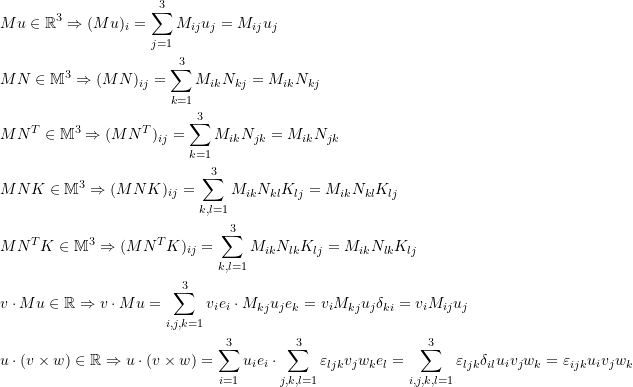### Linear Maps Between Vector Spaces: Einstein Summation Convention

Einstein summation convention is a notational convention in Mathematics that is commonly used in the applications of linear algebra in continuum mechanics. The purpose is to achieve notational brevity. According to Einstein summation convention, when an index appears twice in a single term it implies summation of that term over all the values of the index which are almost always the values of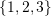since the underlying space is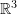. For example, if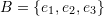is a basis set for, and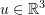, then applying Einstein summation convention implies the following equality: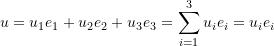The following equalities relating the Kronecker delta, the alternator, and the vectors of the basis set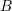are very useful when dealing with continuum mechanics and with Einstein summation convention: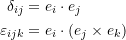These, and using Einstein summation convention can be used to show the following identity: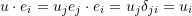Notice that the expression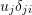is actually summed over the values of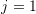to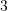. However,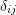is 0 except when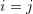and therefore, we are left with only one component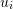. Similarly, if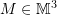,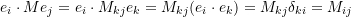The cross product can be simplified using the alternator and the Einstein summation convention as follows. If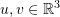, then the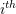component of the vector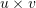has the form: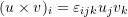For example, the convention can also be used for the following component forms. Let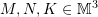, andthen: